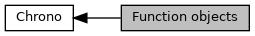Function objects

Description

Collaboration diagram for Function objects:Classes

class  chrono::ChFunction
Interface base class for scalar functions of the type: y= f(x) More...

class  chrono::ChFunction_Const
Constant function: y = C More...

class  chrono::ChFunction_ConstAcc
Constant acceleration function: More...

class  chrono::ChFunction_Derive
Derivative of a function: y = df/dx More...

class  chrono::ChFunction_Fillet3
Cubic fillet function (cubic poly with C0 C1 boundary conditions). More...

class  chrono::ChFunction_Integrate
Integral of a function: y = int{ f(x) dx More...

class  chrono::ChFunction_Lambda< LAMBDA >

class  chrono::ChFunction_Matlab
Matlab function: y = matlab evaluation of function y=f(x) More...

class  chrono::ChFunction_Mirror
Mirror function: y = __/\__ Mirrors a function about a vertical axis. More...

class  chrono::ChFunction_Mocap
Motion capture (sample) function: y = (linear interpolated array of samples) More...

class  chrono::ChFunction_Noise
Noise function: y = multi-octave noise with cubic interpolation. More...

class  chrono::ChFunction_Operation
Operation between functions: More...

class  chrono::ChFunction_Oscilloscope
Oscilloscope function. More...

class  chrono::ChFunction_Poly
POLYNOMIAL FUNCTION: y = a + bx + cx^2 + dx^3 + ... More...

class  chrono::ChFunction_Poly345
Ramp function, as a 3-4-5 polynomial: More...

class  chrono::ChFunction_Ramp
Linear function (like a straight ramp): y = y0 + x * speed More...

class  chrono::ChFunction_Recorder
Recorder function: More...

class  chrono::ChFunction_Repeat
Repeat function: y = __/__/__/ More...

class  chrono::ChFseqNode
Node for the list of functions in a ChFunction_Sequence object. More...

class  chrono::ChFunction_Sequence
Sequence function: y = sequence_of_functions(f1(y), f2(y), f3(y)) All other function types can be inserted into this. More...

class  chrono::ChFunction_Setpoint
Function that returns Y from an externally-provided value, as a ZOH (zero order hold) block. More...

class  chrono::ChFunction_SetpointCallback
Interface for functions that uses a callback to return a Y value, as a ZOH (zero order hold) block. More...

class  chrono::ChFunction_Sigma
Sigma function: y = polynomial smooth ramp More...

class  chrono::ChFunction_Sine
Sine function y = sin (phase + w*x ) where w=2*PI*freq. More...

class  chrono::ChFunctionPosition
Interface base class for scalar->vector functions of the type: More...

class  chrono::ChFunctionPosition_line
A motion function p=f(s) where p(t) is defined with a ChLine geometry object, ex. More...

class  chrono::ChFunctionPosition_setpoint
A motion function p=f(s) where p(t) is an externally-provided sample, as a ZOH (zero order hold) of FOH (first order). More...

class  chrono::ChFunctionPosition_XYZfunctions
A motion function p=f(s) where p(t) is defined with three independent ChFunction objects, each for px, py, pz component. More...

class  chrono::ChFunctionRotation
Interface base class for scalar->quaternion functions of the type: More...

class  chrono::ChFunctionRotation_ABCfunctions
A rotation function q=f(s) where q(s) is defined with three ChFunction objects, each per an an angle in an intrinsic triplets of angles (ex. More...

class  chrono::ChFunctionRotation_axis
A rotation function q=f(s) where q(s) is defined with axis V and angle alpha, assuming fixed axis of rotation V and a angle of rotation alpha about that axis, expressed with a ChFunction object alpha=alpha(s). More...

class  chrono::ChFunctionRotation_setpoint
A rotation q=f(s) provided from a rotation sample, continuously updated by the user, behaving as a ZOH (zero order hold) of FOH (first order). More...

class  chrono::ChFunctionRotation_spline
A rotation function q=f(s) that interpolates n rotations using a "quaternion spline" of generic order. More...

A rotation function q=f(s) that interpolates n rotations using a SQUAD spherical quadrangle interpolation between quaternions. More...

Functions

template<typename T >
ChFunction_Lambda< T > chrono::make_ChFunction_Lambda (T &&func)
Create a stack-allocated ChFunction_Lambda. More...

template<typename T >
std::shared_ptr< ChFunction_Lambda< T > > chrono::make_shared_ChFunction_Lambda (T &&func)
Create a shared pointer to a heap-allocated ChFunction_Lambda. More...

◆ make_ChFunction_Lambda()

template<typename T >
 ChFunction_Lambda chrono::make_ChFunction_Lambda ( T && func )

Create a stack-allocated ChFunction_Lambda.

Template Parameters
 T Type of generic lambda argument. Not necessary to type manually.
Parameters
 func Generic lambda

◆ make_shared_ChFunction_Lambda()

template<typename T >
 std::shared_ptr > chrono::make_shared_ChFunction_Lambda ( T && func )

Create a shared pointer to a heap-allocated ChFunction_Lambda.

Template Parameters
 T Type of generic lambda argument. Not necessary to type manually.
Parameters
 func Generic lambda